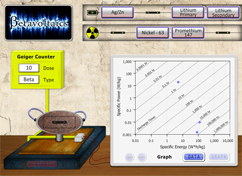# BetavoltaicsHow many times have you used an electronic device and the batteries died? Wouldn’t it be great if batteries could last for 10 or 15 years of normal use? Betavoltaics might be an answer to solving this problem of limited battery life. Get ready to get charged up about this "potential" game-changing technology.

### What is the difference between voltage and current?

Voltage is the energy carried by a charge. It can be measured as the difference in charge between two points of a circuit. Current, on the other hand, is a measurement of how much electricity passes a certain point on the circuit.

### What is the difference between electrical power and electrical energy?

Electrical power is the rate of energy being provided at a specific instance, whereas electrical energy is the amount of power provided over time. Therefore two identical light bulbs would have the capacity to use energy at the same rate and therefore have the same "power" but one may only be on for ten seconds and another for ten hours, so the amount of "energy" used is different.

### How do batteries provide electrical energy?

Batteries produce electricity from a chemical reaction. Inside the battery are a cathode and anode. The cathode is connected to the positive terminal, and the anode is connected to the negative terminal. A separator inside the battery keeps the cathode and anode from touching. When the battery is connected to a circuit, a chemical reaction takes place in both the cathode and the anode. The reaction in the anode releases electrons, and the reaction in the cathode accepts these electrons. To get from the anode to the cathode, the electrons must flow through the circuit, producing electricity. The battery can only supply a current for as long as it still has reactants to undergo the chemical reactions in the anode and cathode. This length of time is called the "discharge time", or the amount of time that it takes the battery to produce all the current that it can.

Some types of atoms have nuclei that are unstable. To become more stable, they decrease their energy by emitting small particles. The atom is said to be radioactive, and the process of emitting these particles is called radioactive decay. Eventually, the radioactive nucleus decays enough to transform into either a stable state, or the nucleus of an entirely different atom. The main types of radioactive decay are alpha decay, beta decay and gamma decay. In alpha decay, alpha particles are emitted from the radioactive nucleus; in beta decay beta particles are emitted, and in gamma decay, gamma rays are emitted. These emissions can be detected by a machine called a Geiger counter, which is used to detect and measure radiation.

### What is beta decay?

Beta decay is a type of radioactive decay where an atom emits a beta particle. A beta particle can either be an electron or a positron (a positron is like an electron, but has a positive charge instead of a negative charge). If the atom emits an electron, the decay is called "beta minus" decay. When the electron is emitted, an electron antineutrino is emitted as well. If the atom emits a positron, it is called "beta plus" decay. When beta plus decay occurs, an electron neutrino is also emitted. By emitting beta particles, the atom can obtain the amount of protons and neutrons that make it most stable.

### How does a betavoltaic source work?

In a betavoltaic source, a beta particle collides with an electron in a semi-conducting material. This collision temporarily moves this electron to a higher energy level and creates a hole in the material that is filled in with another electron. This electron-hole pair formation and filling-in process makes an electrical current. The current produced by betavoltaics is very small and in the nano- or microamps per square centimeter of material range. This is much smaller than the current produced by a photovoltaic cell, which is about 1,000 to 1,000,000 times larger.